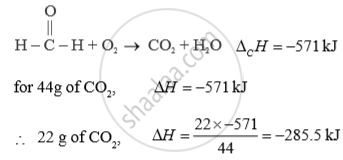Advertisement Remove all ads

# Calculate the Work Done in the Following Reaction at 50o C. State Whether Work is Done on the System Or by the System. - Chemistry

Answer in Brief

Calculate the work done in the following reaction at 50°C. State whether work is done on the system or by the system.
"SO"_2("g") + 1/2"O"_(2("g")) rightarrow "SO"_(3("g"))

Advertisement Remove all ads

#### Solution

"SO"_2("g") + 1/2"O"_(2("g")) rightarrow "SO"_(3("g"))
Δn =  (moles of gaseous product) -  (moles of gaseous reactant)
= 1-(1+1/2)
=-1/2
w = - ΔnRT
-( -1/2 × 8.314 × 323)
=1342.7J
As work done is positive. Therefore work is done by surrounding on system.

(OR)

The standard enthalpy of combustion of formaldehyde Δ 0H0 = -571 kJ How much heat will be evolved in the formation of 22 g of CO2 ?Concept: Chemical Thermodynamics and Energetic - Enthalpy of Bond Dissociation
Is there an error in this question or solution?
Advertisement Remove all ads

#### APPEARS IN

Advertisement Remove all ads

#### Video TutorialsVIEW ALL 

Advertisement Remove all ads
Share
Notifications

View all notifications

Forgot password?